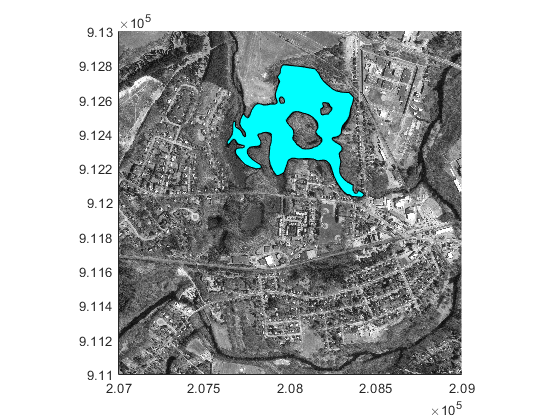mappolyshape

Polygon in planar coordinates

Description

A mappolyshape object represents a polygon or multipolygon in planar coordinates. A polygon is a region bounded by a closed curve and can include interior holes. A multipolygon is an individual polygon shape that includes multiple nonintersecting regions.

To represent a polygon or multipolygon in geographic coordinates, use a geopolyshape object instead.

Creation

To create mappolyshape objects, either:

• Import polygon data in planar coordinates as a geospatial table using the readgeotable function, and then query the Shape variable of the table.

• Use the mappolyshape function (described here).

Description

example

shape = mappolyshape(x,y) creates a mappolyshape object or array of mappolyshape objects with vertices at the specified x- and y-coordinates. The sizes of x, y, and the mappolyshape object array shape match.

The mappolyshape function assumes that x and y define polygons with valid topology. A polygon has valid topology when:

• Region interiors are to the right as you trace boundaries from vertex to vertex.

• The boundaries have no self-intersections.

In general, the outer boundaries of polygons with valid topology have vertices in a clockwise order and the interior holes have vertices in a counterclockwise order.

Input Arguments

expand all

x-coordinates, specified as a numeric vector or a cell array of numeric vectors.

• Create a polygon by specifying a vector, such as [-113 -49 -100 -113].

• Create a polygon with holes or a multipolygon by specifying a vector and including breaks between the hole and region boundaries as NaN values, such as [69 90 105 79 69 NaN 6 52 43 14 6 NaN 18 32 22 18].

• Create an array of polygons and multipolygons by specifying a cell array of vectors, such as {[69 90 105 79 69],[6 52 43 14 6 NaN 18 32 22 18]}.

The NaN values in x must correspond to the NaN values in y.

The size of x must match the size of y. For cell arrays, the size of the vector in each cell of x must match the size of the vector in the corresponding cell of y.

Data Types: double | cell

y-coordinates, specified as a numeric vector or a cell array of numeric vectors.

• Create a polygon by specifying a vector, such as [39 45 19 39].

• Create a polygon with holes or a multipolygon by specifying a vector and including breaks between the hole and region boundaries as NaN values, such as [37 46 31 20 37 NaN 45 49 35 32 45 NaN 35 40 42 35].

• Create an array of polygons and multipolygons by specifying a cell array of vectors, such as {[37 46 31 20 37],[45 49 35 32 45 NaN 35 40 42 35]}.

The NaN values in x must correspond to the NaN values in y.

The size of x must match the size of y. For cell arrays, the size of the vector in each cell of x must match the size of the vector in the corresponding cell of y.

Properties

expand all

Number of regions, returned as an array of nonnegative integers. A region is a connected area such that any two points within the area can be connected by a path entirely within the area. Regions may contain holes.

For a mappolyshape scalar, the value of NumRegions is 1 when the mappolyshape object represents a single polygon and more than 1 when the object represents a multipolygon.

For a mappolyshape array, the size of NumRegions matches the size of the array.

Data Types: double

Number of holes in the polygon or multipolygon, returned as an array of nonnegative integers.

For a mappolyshape array, the size of NumHoles matches the size of the array.

Data Types: double

Geometric type, returned as "polygon".

Data Types: string

Coordinate system type, returned as "planar".

Data Types: string

Projected coordinate reference system (CRS), specified as a projcrs object. A projected CRS consists of a geographic CRS and several parameters that are used to transform coordinates to and from the geographic CRS.

Examples

collapse all

Import a shapefile containing hydrographic data for Concord, MA as a geospatial table. The shapefile represents the data using polygons.

Create a subtable that contains a polygon representing a pond. Get information about the polygon by querying the Shape variable of the table.

pond = hydro(14,:);
pond.Shape
ans =
mappolyshape with properties:

NumRegions: 1
NumHoles: 3
Geometry: "polygon"
CoordinateSystemType: "planar"
ProjectedCRS: [1×1 projcrs]

Import an orthophoto of Concord, MA, along with a map cells reference object and a colormap. Display the orthophoto and polygon on a map.

mapshow(ortho,cmap,R)
mapshow(pond,FaceColor="c")Create an individual polygon with no holes as a mappolyshape scalar. Specify the projected CRS as World Equidistant Cylindrical, which has the EPSG authority code 4087.

x = [-113 -49 -100 -113];
y = [39 45 19 39];
polyshp = mappolyshape(x,y);

p = projcrs(4087);
polyshp.ProjectedCRS = p
polyshp =
mappolyshape with properties:

NumRegions: 1
NumHoles: 0
Geometry: "polygon"
CoordinateSystemType: "planar"
ProjectedCRS: [1x1 projcrs]

Create a multipolygon with two regions and one hole as a mappolyshape scalar.

x = [69 90 105 79 69 NaN 6 52 43 14 6 NaN 18 32 22 18];
y = [37 46 31 20 37 NaN 45 49 35 32 45 NaN 35 40 42 35];
multipolygon = mappolyshape(x,y);
multipolygon.ProjectedCRS = p
multipolygon =
mappolyshape with properties:

NumRegions: 2
NumHoles: 1
Geometry: "polygon"
CoordinateSystemType: "planar"
ProjectedCRS: [1x1 projcrs]

Create two individual polygons as a 1-by-2 mappolyshape array. The second polygon contains a hole.

x = {[69 90 105 79 69],[6 52 43 14 6 NaN 18 32 22 18]};
y = {[37 46 31 20 37],[45 49 35 32 45 NaN 35 40 42 35]};
polyArray = mappolyshape(x,y);
polyArray.ProjectedCRS = p
polyArray=1×2 object
1x2 mappolyshape array with properties:

NumRegions: [1 1]
NumHoles: [0 1]
Geometry: "polygon"
CoordinateSystemType: "planar"
ProjectedCRS: [1x1 projcrs]

Topics

Introduced in R2021b

Mapping Toolbox Documentation